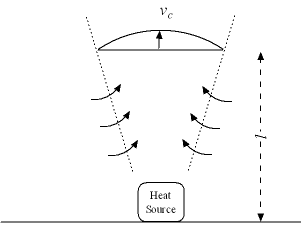Engineering ToolBox - Resources, Tools and Basic Information for Engineering and Design of Technical Applications!

# Convective Air Flow - Single Heat Source

## Calculate the vertical air flow and air velocity generated by a single heat source.

A heat source, like an engine, stove, melting pot or a person, will generate a convective vertical air flow as indicated in the figure below.### Air Velocity

The air velocity in the center of the air flow at a distance above the floor can be calculated as

vc = c1 (P / l)1/3                                              (1)

where

vc = air velocity in center of the air flow (m/s)

c1 = constant characterizing the actual application, typical values ranging 1 to 2

P = heat power from the source (kW)

l = distance above the floor and the heat source (m)

### Air Flow Volume

The air flow volume in a distance above the the floor can be calculated as

Q = c2 P1/3 l5/3                                       (2)

where

Q = air flow volume (m3/s)

c2 = constant characterizing the actual application, values ranging 0.05 to 0.15 (typical 0.06)

### Example - Convective Air Flow above an Engine

The heat loss from the surface of an engine is 10 kW. If c1 = 1.5, the air velocity 3 meters above the engine can be estimated to

vc = 1.5 ((10 kW) / (3 m))1/3

= 2.2 m/s

With c2 = 0.06 the volume flow can be estimated to

Q = 0.06 (10 kW)1/3 (3 m)5/3

= 0.8 m3/s

## Related Topics

• ### Heating

Heating systems - capacity and design of boilers, pipelines, heat exchangers, expansion systems and more.

## Related Documents

• ### Convective Air Flows from Typical Heat Sources

Convective air flows from typical heat sources like people, computers, radiators and more.
• ### Convective Heat Transfer

Heat transfer between a solid and a moving fluid is called convection. This is a short tutorial about convective heat transfer.
• ### Convective Heat Transmission - Air Velocity and Air Flow Volume

Hot or cold vertical surfaces generates vertical air flows - calculate air velocity and volume flow.
• ### Exhaust Hoods

Sizing of exhaust hoods - air volume flow and capture velocities - online exhaust hood calculator.

## Search

Search is the most efficient way to navigate the Engineering ToolBox.

## Engineering ToolBox - SketchUp Extension - Online 3D modeling!

Add standard and customized parametric components - like flange beams, lumbers, piping, stairs and more - to your Sketchup model with the Engineering ToolBox - SketchUp Extension - enabled for use with older versions of the amazing SketchUp Make and the newer "up to date" SketchUp Pro . Add the Engineering ToolBox extension to your SketchUp Make/Pro from the Extension Warehouse !

We don't collect information from our users. More about

## Citation

• The Engineering ToolBox (2005). Convective Air Flow - Single Heat Source. [online] Available at: https://www.engineeringtoolbox.com/convective-heat-source-d_1007.html [Accessed Day Month Year].

Modify the access date according your visit.

12.1.10